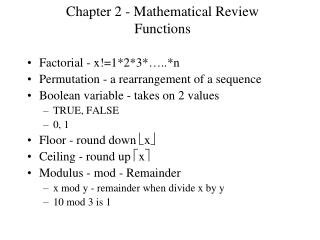DownloadDownload PresentationChapter 2 - Mathematical Review Functions

# Chapter 2 - Mathematical Review Functions

Download Presentation## Chapter 2 - Mathematical Review Functions

- - - - - - - - - - - - - - - - - - - - - - - - - - - E N D - - - - - - - - - - - - - - - - - - - - - - - - - - -
##### Presentation Transcript

1. Chapter 2 - Mathematical ReviewFunctions • Factorial - x!=1*2*3*…..*n • Permutation - a rearrangement of a sequence • Boolean variable - takes on 2 values • TRUE, FALSE • 0, 1 • Floor - round down x • Ceiling - round up x • Modulus - mod - Remainder • x mod y - remainder when divide x by y • 10 mod 3 is 1

2. Logarithms • logby=x  bx=y  blogby=y • log mn = log m + log n • XAXB=XA+B • log m/n = log m - log n • XA/XB=XA-B • log nr = r log n • (XA)B=XAB • logan=logbn/logba • 2n+2n=2*2n=2n+1

3. Recursion • A recursive algorithm calls itself. • Must have a base case to stop recursion • Calls must pass a “smaller problem” to the function. This will cause the recursion to eventually stop. • Factorial example: int fact(int n) { if (n<=1) return 1; else return n*fact(n-1) } • Looks a bit like induction • Better to use for’s and while’s when appropriate.

4. Summations • Adding up a list of values • f(1)+f(2)+f(3)+…+f(n) • if f(i)=i then sum is n(n+1)/2 • if f(i)=i2 then sum is n(n+1)(2n+1)/6 • if f(i)=2i then sum is 2n+1–1 • if f(i)=Ai then sum is (An+1 –1)/(A-1)

5. Recurrence Relations • Many of our operations (functions) will be recursive. • We will want to analyze the running time for a problem of size n. • Recursion defines this in terms of some work plus the amount of time to solve a smaller problem (using the same algorithm). • A mathematical representation of this time will look like: • T(n) = T(n-1) + 1 • Need the time for the base case • T(1) = 0

6. Recurrence Relation Solving • Many recurrence relations are solved using the brute force method. Expand it; see the pattern; solve it. • For example: • T(n) = T(n-1)+1 = T(n-1)+1+1 = T(1)+(n-1) = n-1 • Another example: • T(n) = T(n-1)+n; T(1)=1 • T(n) = T(n-1)+n = T(n-2)+(n-1)+n = T(1)+2+…n = 1+2+3…+n = n(n+1)/2

7. Proof Techniques • Proof by Contradiction • Find a counterexample to prove theorem is false • Assume the opposite of the conclusion is true. Use steps to prove a contradiction (for example 1=2), then the original theorem must be true. • Proof by induction • Show the theorem holds for a base case (n=0 or n=1) • Assume the theorem holds for n=k-1 • Prove the theorem is true for n=k • Example: show 1+2+3+…+n=n(n+1)/2 • for n=1 1=1(2)/2=1 • assume 1+2+3+…+n-1=(n-1)(n)/2; show 1+2+…+n=n(n+1)/2 • (n-1)(n)/2+n=[(n-1)(n)+2n]/2=[n(n-1+2)]/2=n(n+1)/2